In-Place Computation

# In-Place Computation - Notes | Study Signals and Systems - Electronics and Communication Engineering (ECE)

 1 Crore+ students have signed up on EduRev. Have you?

This efficient use of memory is important for designing fast hardware to calculate the FFT. The term in-place computation is used to describe this memory usage.

Decimation in Time Sequence

In this structure, we represent all the points in binary format i.e. in 0 and 1. Then, we reverse those structures. The sequence we get after that is known as bit reversal sequence. This is also known as decimation in time sequence. In-place computation of an eight-point DFT is shown in a tabular format as shown below −

POINTSBINARY FORMATREVERSALEQUIVALENT POINTS
00000000
10011004
20100102
30111106
41000011
51011015
61100113
71111117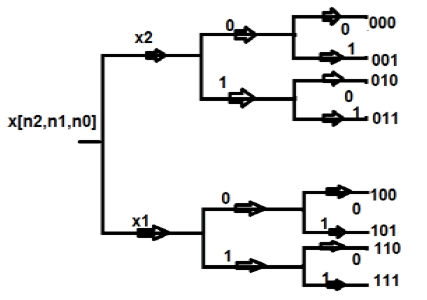Decimation in Frequency Sequence

Apart from time sequence, an N-point sequence can also be represented in frequency. Let us take a four-point sequence to understand it better.

Let the sequence be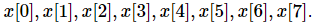We will group two points into one group, initially. Mathematically, this sequence can be written as;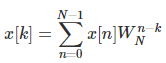Now let us make one group of sequence number 0 to 3 and another group of sequence 4 to 7. Now, mathematically this can be shown as;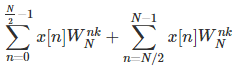Let us replace n by r, where r = 0, 1 , 2….(N/2-1). Mathematically,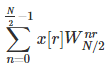We take the first four points (x, x, x, x) initially, and try to represent them mathematically as follows −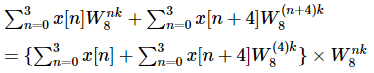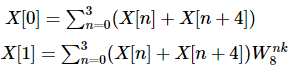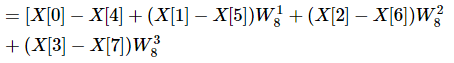We can further break it into two more parts, which means instead of breaking them as 4-point sequence, we can break them into 2-point sequence.

The document In-Place Computation - Notes | Study Signals and Systems - Electronics and Communication Engineering (ECE) is a part of the Electronics and Communication Engineering (ECE) Course Signals and Systems.
All you need of Electronics and Communication Engineering (ECE) at this link: Electronics and Communication Engineering (ECE)

## Signals and Systems

32 videos|76 docs|63 tests
 Use Code STAYHOME200 and get INR 200 additional OFF

## Signals and Systems

32 videos|76 docs|63 tests

Track your progress, build streaks, highlight & save important lessons and more!

,

,

,

,

,

,

,

,

,

,

,

,

,

,

,

,

,

,

,

,

,

;